Open in App
Not now

# Class 12 RD Sharma Solutions – Chapter 21 Areas of Bounded Regions – Exercise 21.1 | Set 2

• Last Updated : 20 May, 2021

### Question 11. Sketch the region {(x, y) : 9x2 + 4y2 = 36} and find the area enclosed by it, using integration.

Solution:

9×2 + 4y2 = 36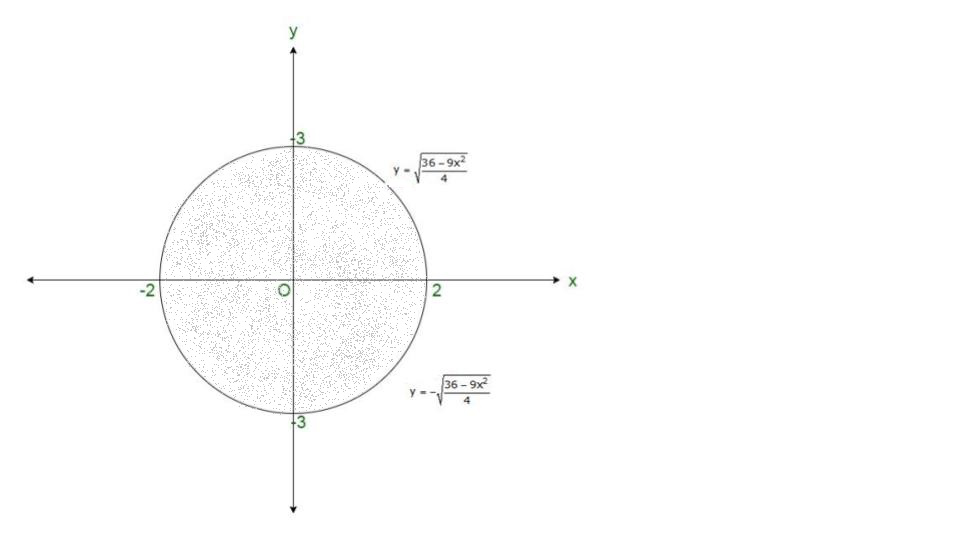Area of Sector OABCO =Area of the whole figure = 4 x area of DOABCO### Question 12. Draw a rough sketch of the graph of the function, x ∈ [0, 1] and evaluate the area enclosed between the curve and the x-axis

Solution:

Here, we have to find the area enclosed between the curve and x-axis.Equation (1) represents an ellipse with centre at origin and passes through (±1, 0) and (0, ±2) and x ∈ [0, 1] as represented by region between y-axis and line x = 1.

Here, is the rough sketch.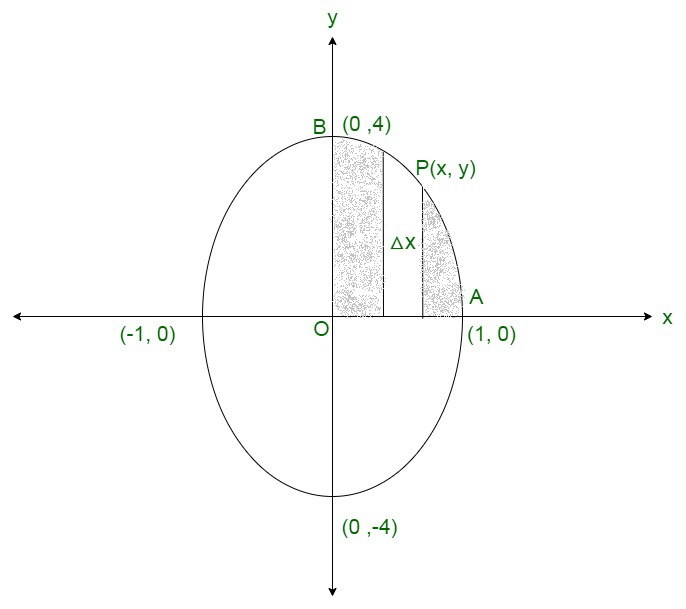Shaded region represents the required area.

We slice it into approximation rectangle of

Width = △x

Length = y

Area of rectangle = y△x

The approx rectangles slide from x = 0 to x = 1,

Thus,

Required area = Region OAPBORequired area =square units

### Question 13. Determine the area under the curveincluded between the line x = 0 and x = 8.

Solution:

Here,

We have to find area under the curvex2 + y2 = a  ………..(1)

between x = 0    ………(2)

x = a    ………..(3)

Equation (1) represents a circle with Centre (0, 0) and passes axes at (0, ±a), (±a, 0).

Equation (2) represents y-axis and

Equation x = a represents  a line parallel to y-axis passing through (a, 0)

Here, is the rough sketch,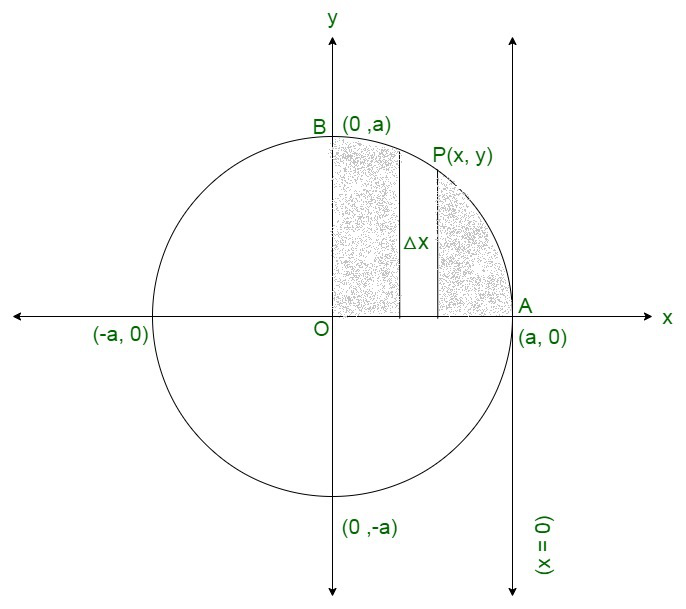Shaded region represents the required area.

We slice it into approximation rectangle of

Width = △x

Length = y

Area of rectangle = y△x

The approx rectangles slide from x = 0 to x = a,

Thus,

Required area = Region OAPBORequired area =square units

### Question 14. Using integration, find the area of the region bounded by the line 2y = 5x + 7, x-axis and the lines x = 2 and x = 8

Solution:

Here,

We have to find area bounded by x-axis

2y + 5x = 7   ………(1)

x = 2      ……..(2)

x = 8    ………(3)

Equation (1)  represents line passing throughandequation.

Equation (2), (3) shows line parallel to y-axis passing through (2, 0), (8, 0) respectively.

Here, is the rough sketch;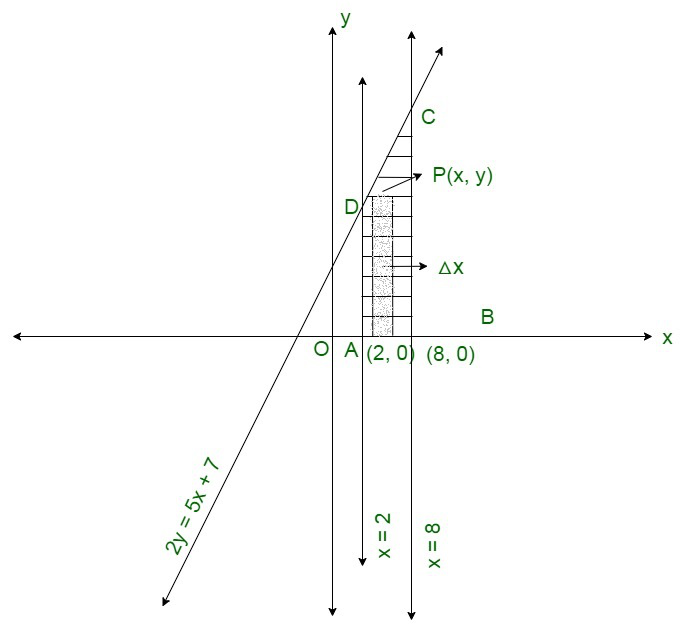Shaded region represents the required area.

We slice it into approximation rectangle of

Width = △x

Length = y

Area of rectangle = y△x

The approx rectangles slide from x = 2 to x = 8,

Thus,

Required area = Region ABCDARequired area = 96 square units

### Question 15. Using definite integrals, find the area of the circle x2 + y2 = a2

Solution:

Here, we have to find the area of circle,

x2 + y2 = a2

Equation (1) represents a circle with centre (0, 0) and radius a, Thus is meets the axes (±a, 0), (0, ±a).

Here, is the rough sketch;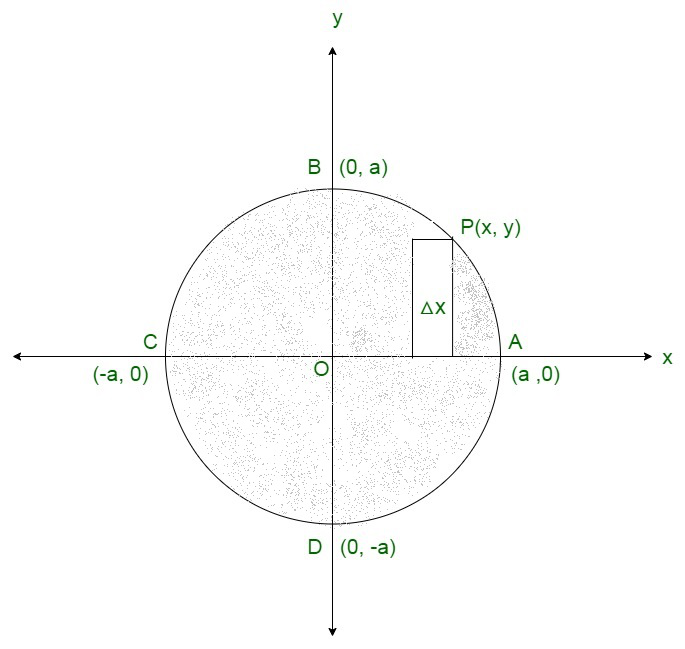Shaded region represents the required area.

We slice it into approximation rectangle of

Width = △x

Length = y

Area of rectangle = y△x

The approx rectangles slide from x = 0 to x = a,

Thus,

Required area = Region ABCDA

= 4 ( Region ABOA)### Question 16. Using the integration, find the area of the region bounded by the following curves, after making a rough sketch: y = 1 + |x + 1|, x = -2, x = 3, y =

Solution:

Here, we have to find the area enclosed by;

x = -2,

x = 3,

y = 0 and

y = 1 + |x + 1|

⇒ y = 1 + x + 1, if x + 1 0

⇒ y = 2 + x    ……….(1), if x ≥ -1

and

⇒ y = 1 – (x + 1), if x + 1 < 0

⇒ y = 1 – x – 1, if x < -1

⇒ y = -x    ………(2), if x < -1

Thus,

Equation (1) is a straight line that passes through (0, 2) and (-1 , 1).

Equation (2) is a line passing through (-1, 1) and (-2, 2) and it is enclosed by line x = 2 and x = 3 which are lines parallel to y-axis and pass through (2, 0) and (3, 0) respectively y = 0 is x-axis

Here is the rough sketch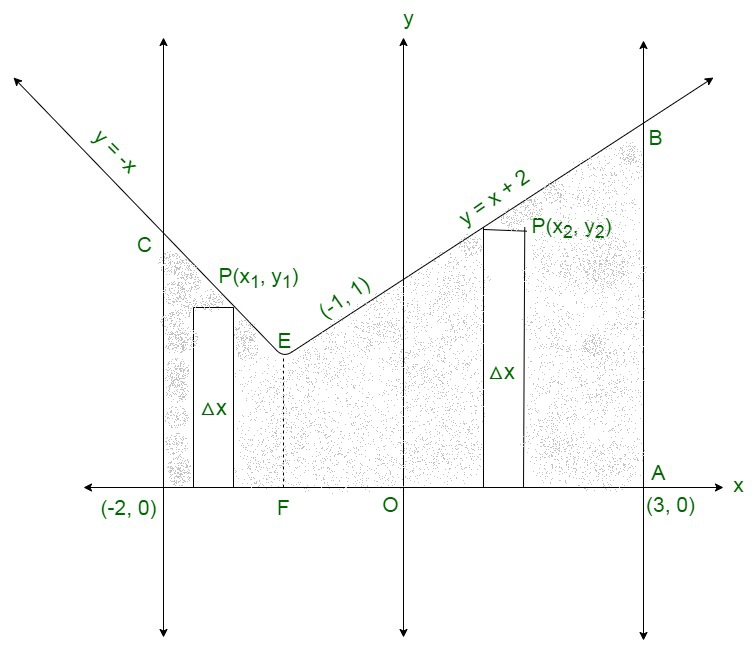Shaded region represents the required area.

Thus,

Required area = Region (ABECDFA)

Required area = (Region ABEFA + Region ECDFE)    ……..(1)

Region ECDFE

We slice it into approximation rectangle of

Width = △x

Length = y1

Area of rectangle = y1△x

The approx rectangles slide from x = -2 to x = -1,

Region ABEFA

We slice it into approximation rectangle of

Width = △x

Length = y2

Area of rectangle = y2△x

The approx rectangles slide from x = -1 to x = 3,

Required area =Required area =square units

### Question 17. Sketch the graph y = |x -5|. Evaluate. What does the value of the integral represent on the graph?

Solution:

Here, is the sketch of the given graph:

y = |x – 5|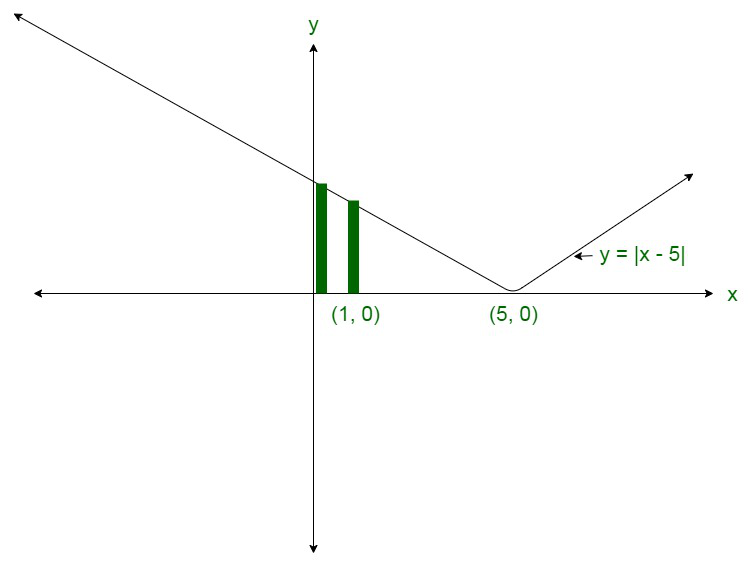Hence,

Required area =Thus,

The given integral represents the area bounded by the curves that are,

x = 0,

y = 0,

x = 1

and

y = -(x – 5).

### Question 18. Sketch the graph of y = |x + 3| and evaluate. What does this integral represents on the graph?

Solution:

Here,

The given equation is y = |x + 3|

The corresponding values of x and y are given in the following table.

Thus,

After plotting these points,

We will get the graph of y = |x + 3|

It is shown as;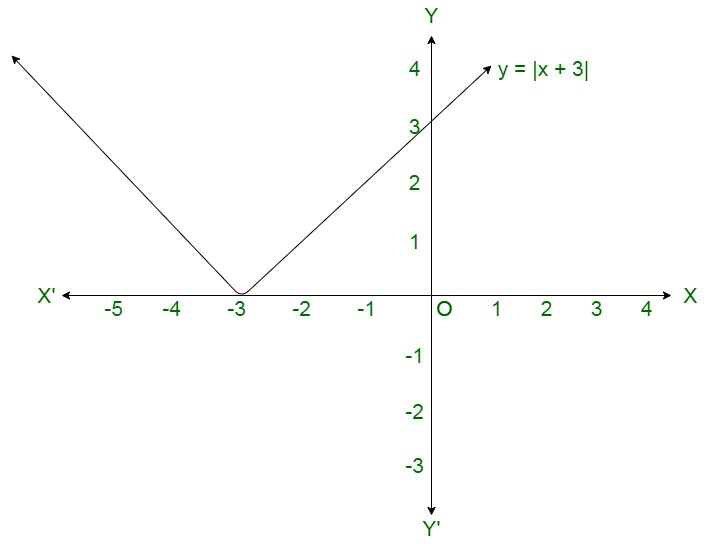It is known that (x + 3) ≤ 0 for -6 ≤ x ≤  -3 and (x + 3) ≥ 0 for -3 ≤ x ≤ 0

Therefore,Question 19. Sketch the graph y = |x + 1|. Evaluate. What does the value of this integral represent on this graph?

Solution:

Here,

Given:

y = |x + 1|=y = x + 1   …………(1)

and

y = -x – 1   ……….(2)

Equation (1) represents a line which meets axes at (0, 1).

Equation (2) represents a line passing through (0, -1) and (-1, 0)

Here is the rough sketch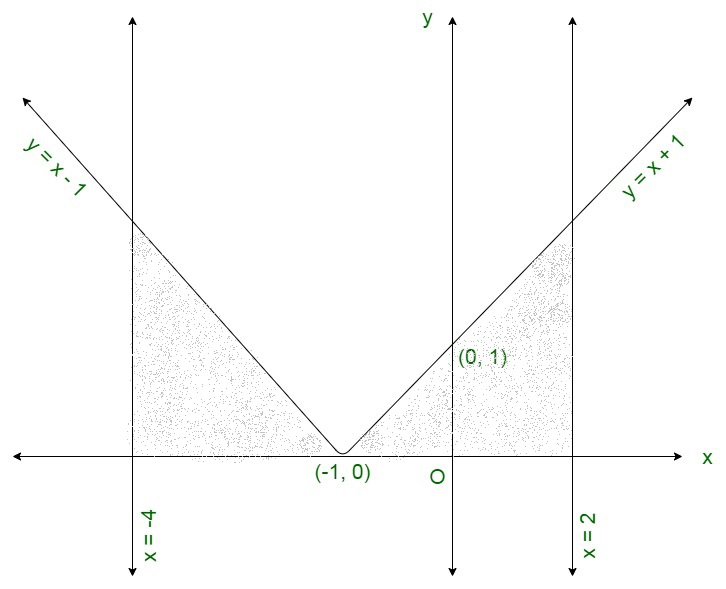Required area = 9 square units.

### Question 20. Find the area of the region bounded by the curve xy – 3x – 2y – 10 = 0, x-axis and the lines x = 3, x = 4.

Solution:

Here,

We have to find the area bounded by

x axis,

x = 3,

x = 4

and

xy – 3x -2y – 10 = 0

⇒ y(x – 2) = 3x + 10

⇒Here, is the rough sketch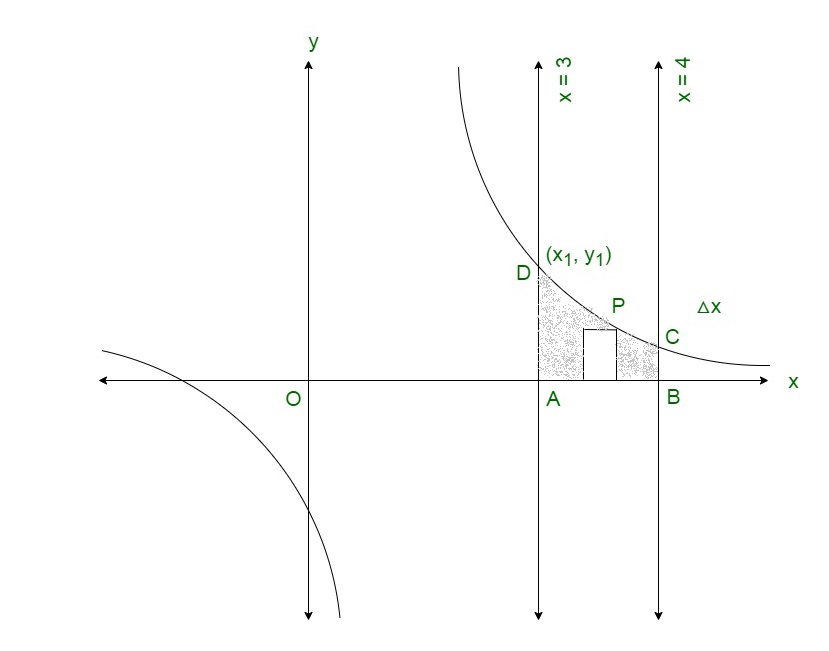Shaded region represents the required area.

We slice it into approximation rectangle of

Width = △x

Length = y

Area of rectangle = y△x

The approx rectangles slide from x = 3 to x = 4,

Required area = Region ABCDARequired area = (3 + 16 log2) square units.

My Personal Notes arrow_drop_up